﻿ 一种新的利用单基站GNSS反演大气剖面的技术文章快速检索 高级检索
 大地测量与地球动力学2019, Vol. 39Issue (12): 1283-1286,1303  DOI: 10.14075/j.jgg.2019.12.013### 引用本文E Shenglong, LUO Yingting, WU Hao, et al. A New Technique for Profiling Tropospheric Refractivity Based on Single Ground-Based GNSS Receiver[J]. Journal of Geodesy and Geodynamics, 2019, 39(12): 1283-1286,1303.### Foundation support

Science and Technology Project of China Southern Power Grid Guangdong Power Grid Co Ltd, No.036100KK52170058; National Key Research and Development Program of China, No. 2018YFC0823704; China Postdoctoral Science Foundation, No. 2019M652706; Fundamental Research Funds for the Central Universities, No.2042019kf0032.

### Corresponding author

XIA Pengfei, PhD, assistant researcher, majors in GNSS meteorology, E-mail:pfxia130@whu.edu.cn.

### 第一作者简介

E Shenglong, engineer, majors in application research of Beidou positioning technology in power industry, E-mail:esloong@126.com.

### 文章历史

1. 广东电网公司电力科学研究院，广州市东风东路水均岗8号，510080;
2. 武汉大学卫星导航定位技术研究中心, 武汉市珞喻路129号，430079

Lowry等首次提出基于地基单GNSS测站获得的斜路径延迟来反演对流层大气折射率剖面。随后，林乐科等[3-6]对单站地基GNSS探测大气折射率剖面进行深入研究，实现了基于对流层天顶延迟的折射率剖面反演，并在广东东南沿海地区开展单站地基GNSS探测大气的大型观测实验。目前，单站地基GNSS反演大气折射率的模型仍需进一步改进，以提高大气折射率的反演精度。本文提出一种新的利用天顶对流层延迟反演大气折射率的模型，并选用中国及周边区域8个IGS测站的观测数据进行试算。将测站附近探空产品获取的大气折射率作为真值，统计结果显示，与传统的反演模型相比，新的反演模型提高了大气折射率的反演精度。

1 传统的基于天顶对流层延迟的模型参数反演

1.1 干项模型参数估计

 $N_T^h \approx N_T^{{h_0}}{\left( {\frac{{{T_0} + \beta h}}{{{T_0}}}} \right)^{ - \left( {1 + \frac{g}{{\beta {R_d}}}} \right)}}$ (1)

ZHD可表示为干项折射率的积分

 ${\rm{ZHD}} = {10^{ - 6}}\int_{{h_0}}^{{H_h}} {N_T^h} (h){\rm{d}}h$ (2)

 $\begin{array}{*{20}{c}} {{\rm{ZH}}{{\rm{D}}^{{\rm{Tro}}}} = {{10}^{ - 6}}\int_0^{{H_{{\rm{Tro}}}}} {N_T^h} (h){\rm{d}}h = }\\ {{{10}^{ - 6}}N_T^{{h_0}}\frac{{{{\left( {{T_0} + \beta {H_{{\rm{Tro}}}}} \right)}^{\eta + 1}} - T_\delta ^{\eta + 1}}}{{(\eta + 1)T_0^\eta \beta }}} \end{array}$ (3)

1.2 湿项模型参数估计

 $Z{\rm{WD}} = Z{\rm{TD}} - Z{\rm{HD}}$ (4)

ZWD可表示为湿项折射率Nw的积分：

 $Z{\rm{WD}} = {10^{ - 6}}\int_0^\infty {{N_w}} (h){\rm{d}}h$ (5)

Nw随高程呈现近似指数形式的分布

 ${N_w}\left( h \right) = N_w^0\exp \left( {\frac{{ - h}}{{{H_{we}}}}} \right)$ (6)

 $Z{\rm{WD}} = {10^{ - 6}}{H_{we}}N_w^0$ (7)

2 新的基于天顶对流层延迟的模型参数反演 2.1 新的干项模型参数估计

ERA-Intrim提供了不同高程上的压强、温度、湿度等气象产品，利用这些产品可以计算出不同高程上的大气干项折射率及ZHD：

 $N_T^h = 77.6 \cdot \frac{{{P_h}}}{{{T_h}}}$ (8)
 ${\rm{ZH}}{{\rm{D}}_h} = \frac{{{{10}^{ - 6}} \cdot \sum\limits_{i = 0}^{i = n - 1} {\left( {N_T^{{h_i}} + N_T^{{h_{i + 1}}}} \right)} }}{2}$ (9)

 ${\rm{RZH}}{{\rm{D}}_h} = \frac{{{\rm{ZH}}{{\rm{D}}_h}}}{{{\rm{ZH}}{{\rm{D}}_1}}}$ (10)

2.2 新的湿项模型参数估计

 $\left( {\frac{{N_h^w}}{{N_{{h_0}}^w}}} \right) = {\left( {\frac{{P_w^h}}{{P_w^{{h_0}}}}} \right)^\gamma } = {\left( {\frac{{{T_0} + \beta \left( {h - {h_0}} \right)}}{{{T_0}}}} \right)^{\frac{{\gamma {g_0}}}{{\beta {R_d}}}}}$ (11)

 $\begin{array}{*{20}{c}} {Z{\rm{WD}} = {{10}^{ - 6}} \cdot N_{{h_0}}^w\int_0^\infty {{{\left( {\frac{{{T_0} + \beta \left( {h - {h_0}} \right)}}{{{T_0}}}} \right)}^\gamma }} {\rm{d}}h = }\\ {{{10}^{ - 6}} \cdot N_{{h_0}}^w \cdot \frac{{{T_0}\left( {E + 1} \right)}}{\beta } \cdot }\\ {\left[ {1 - {{\left[ {\frac{{{T_0} + \beta \left( {{H_{{\rm{top}}}} - {h_0}} \right)}}{{{T_0}}}} \right]}^{\left( {E + 1} \right)}}} \right]} \end{array}$ (12)

3 结果分析 3.1 数据来源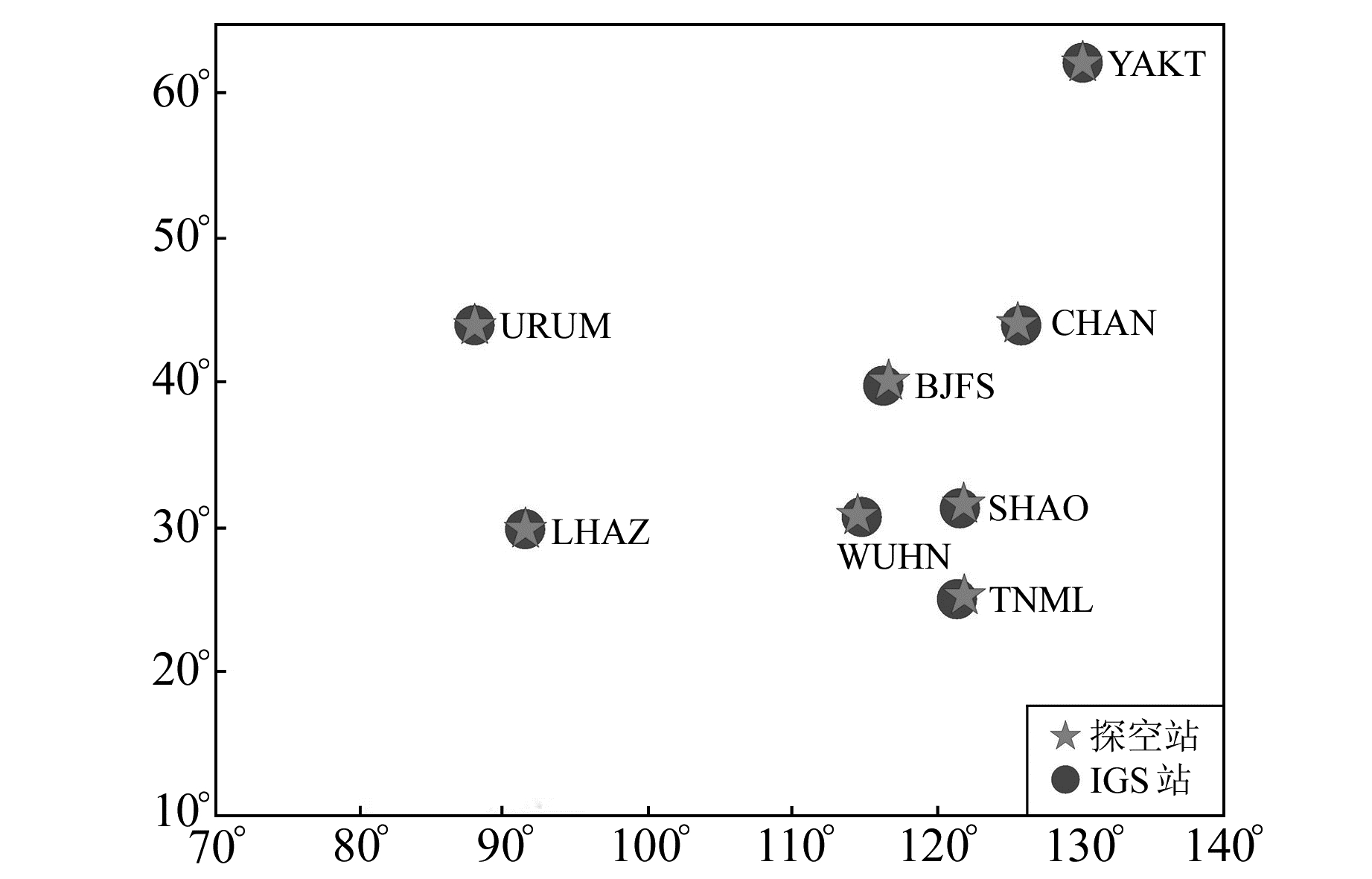图 1 所选取的8个IGS测站及附近探空测站分布 Fig. 1 Distribution of the selected eight IGS stations and nearby radiosonde stations
3.2 确定HTroHtop

Era-Interim提供高垂直分辨率的大气压强、大气温度和湿度等大气产品，可从网站http://apps.ecmwf.int/datasets/data/interim-full-daily/下载。选择中国地区2006~2016-07的Era-Interim产品，分别利用式(10)和MWO方法估算出HTroHtop，最后分别利用传统和新的模型参数反演出大气折射率。表 1给出中国及周边区域8个IGS测站2016-07的HTroHtop表 1 8个IGS测站2016-07的HTroHtop Tab. 1 HTro and Htop of eight IGS staions in July, 2016

3.3 反演结果与探空数据的比较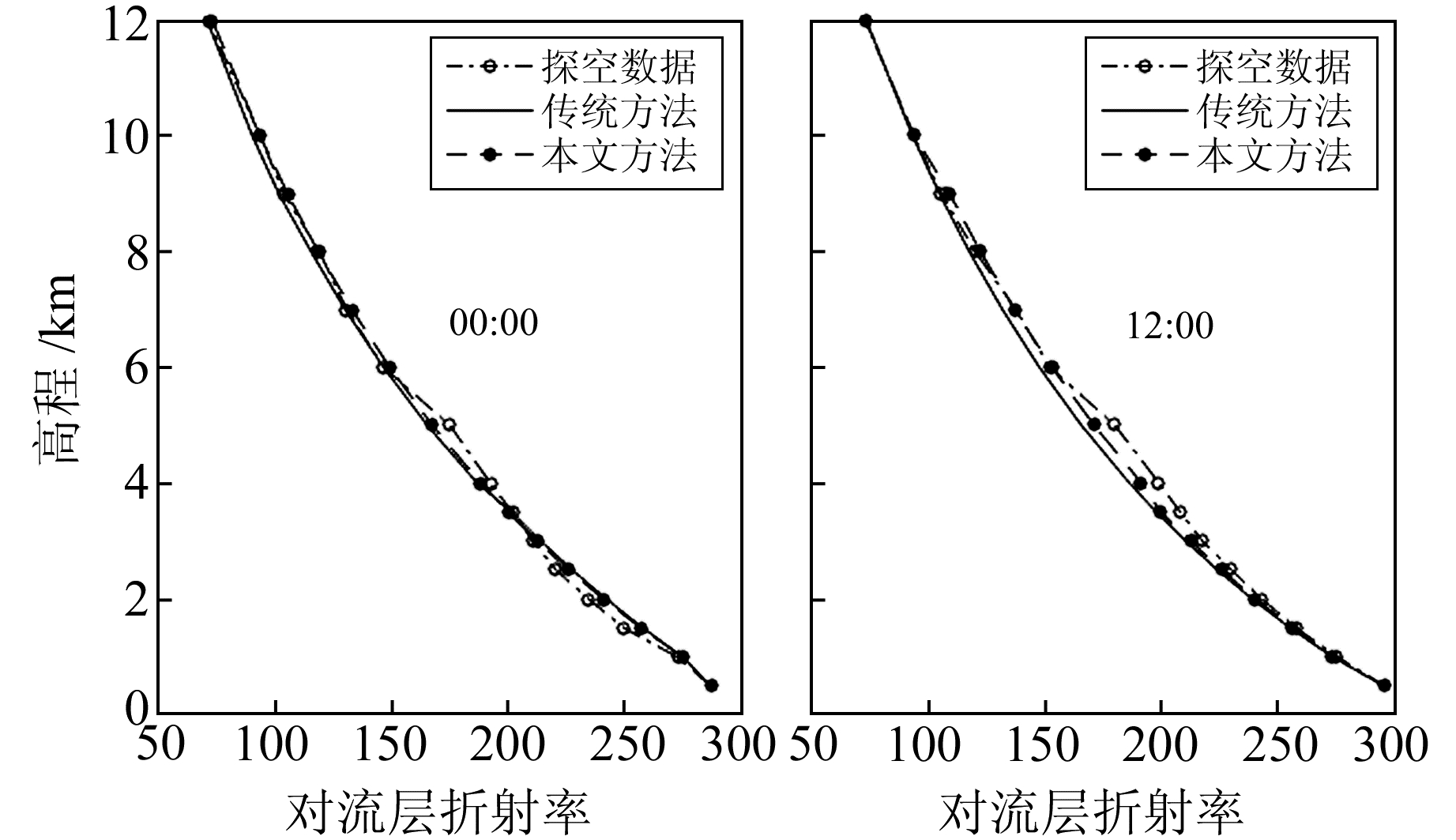图 2 URUM站2016年第183天反演结果 Fig. 2 The inversion at URUM station on doy 183 of 2016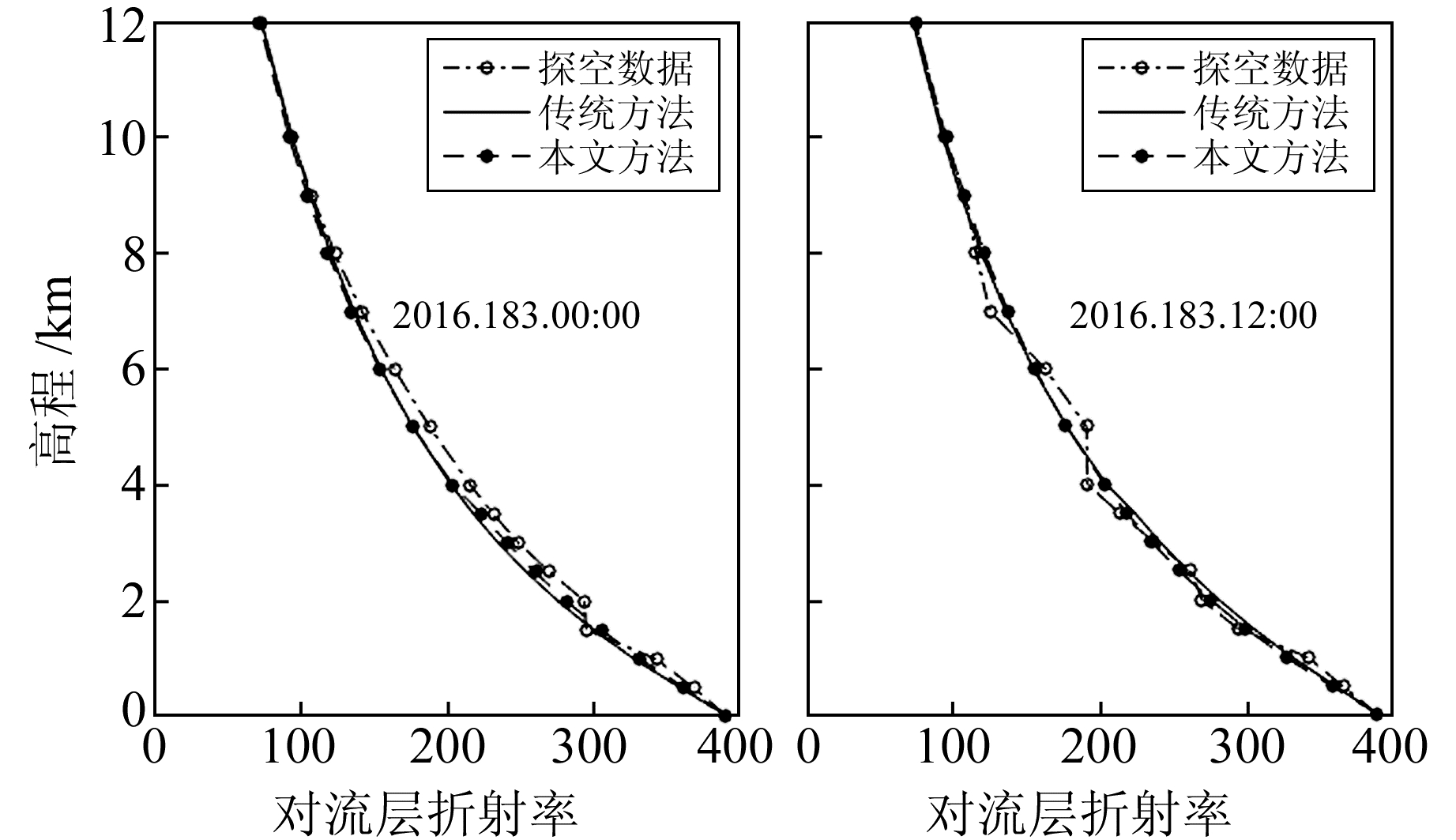图 3 WUHN站2016年第183天反演结果 Fig. 3 The inversion at WUHN station on doy 183 of 2016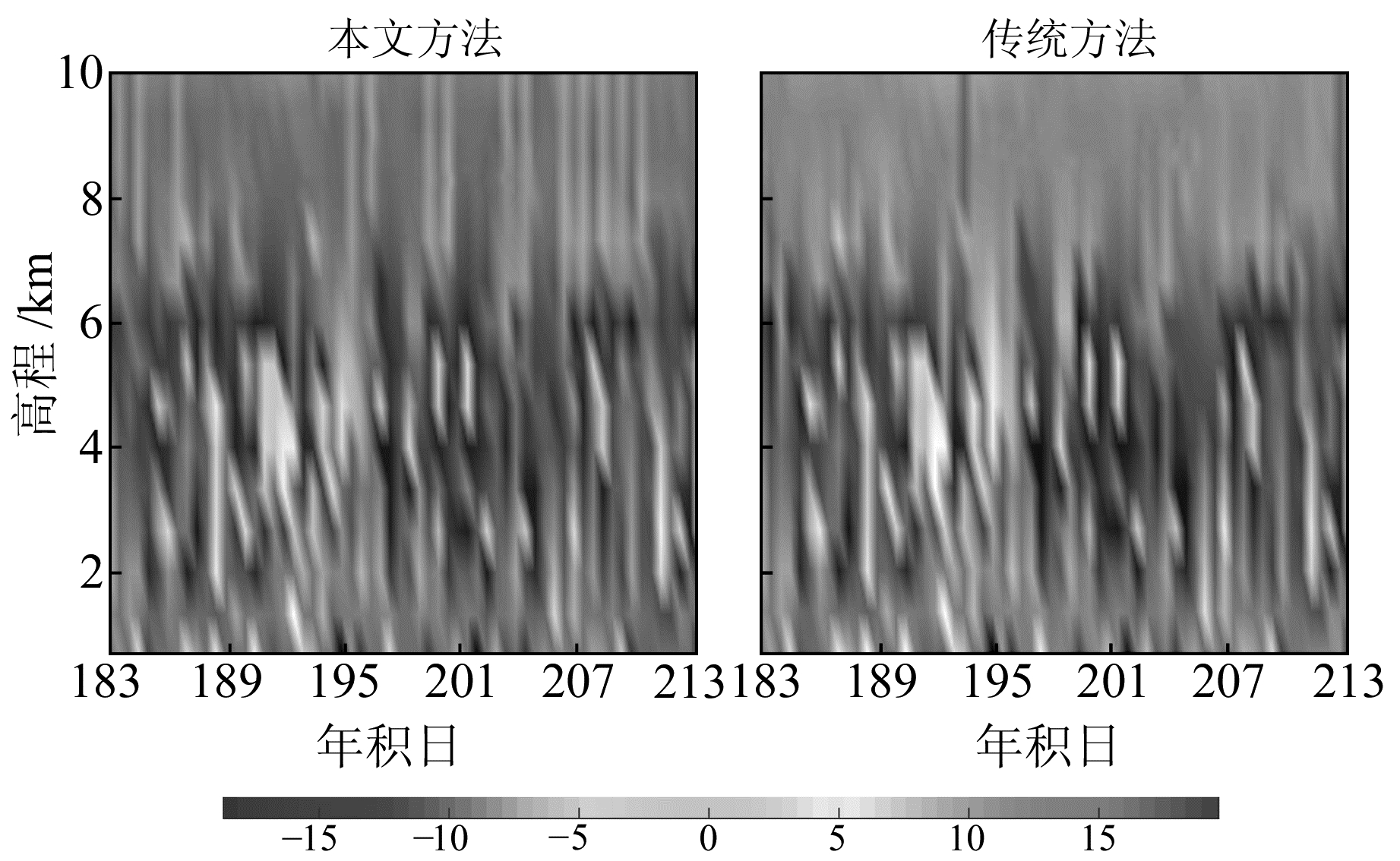图 4 URUM站2016-07模型参数反演结果与真值的偏差 Fig. 4 The deviation of inversion between model-derived and true values at URUM station in July 2016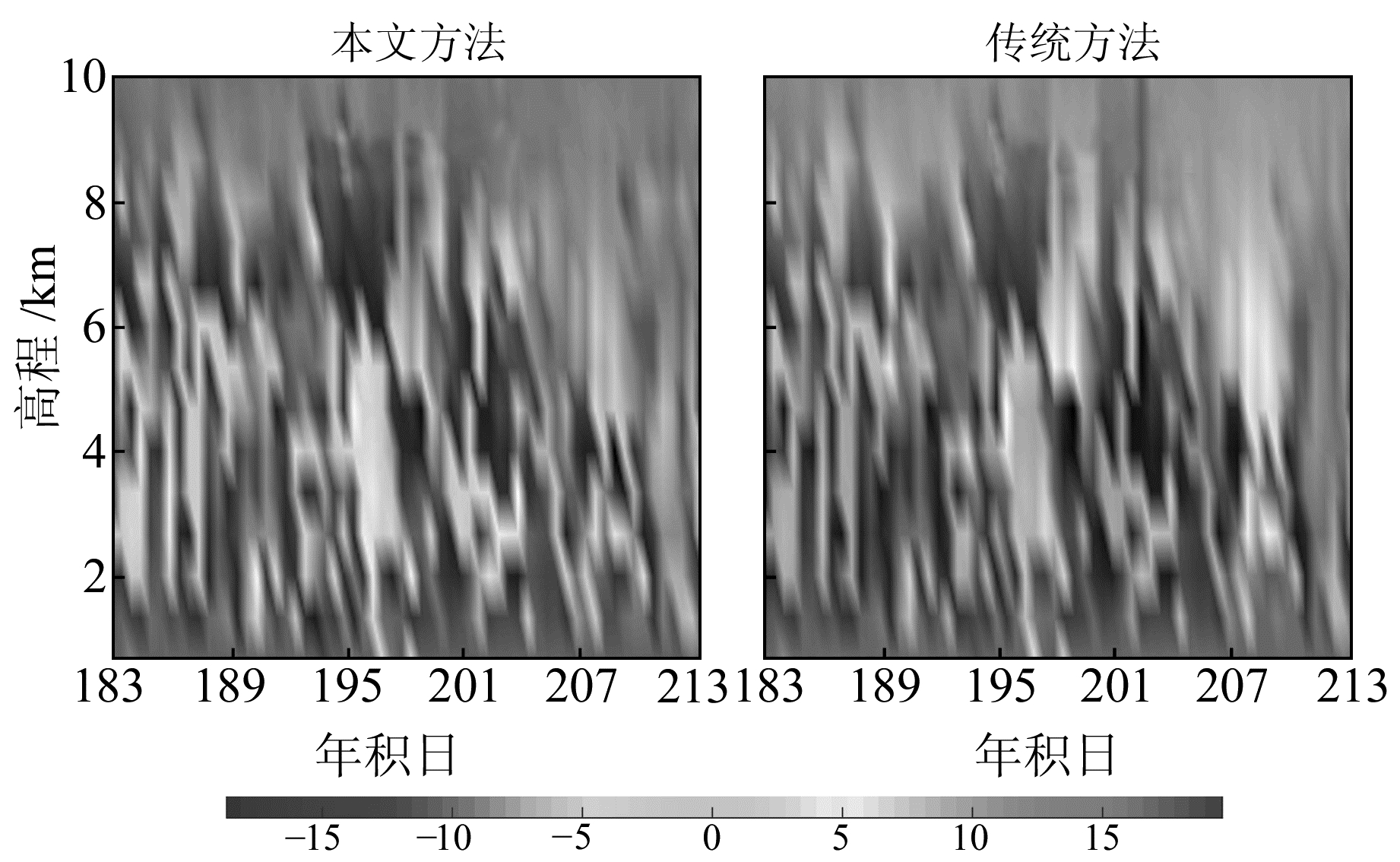图 5 WUHN站2016-07模型参数反演结果与真值的偏差 Fig. 5 The deviation of inversion between model-derived and true values in WUHN station in July 2016表 2 8个IGS测站模型参数反演的结果与真值之间的偏差 Tab. 2 The statistical values of deviation between model-derived and true values at the eight IGS stations

4 结语

GNSS气象学使得对流层延迟成为反演大气参数的有效信号源。本文利用天顶对流层延迟值，基于模型参数法反演不同高程处的大气折射率，并利用探测产品获得的大气折射率作为真值来评估新的模型参数法的可行性。选择中国及周边地区8个IGS测站2016-07的观测数据进行试算，结果显示，与传统的模型参数法反演的结果相比，新的模型参数法反演的大气折射率更接近真值。然而，当大气折射率的空间分布中存在“逆增层”现象时，2种模型参数法获得的结果都与真值存在较大的偏差。如何反演大气折射率“逆增层”现象将是下一步重点研究的内容。

  林乐科, 张业荣, 赵振维, 等. 基于支持向量机的地基单站GPS反演大气剖面[J]. 南京邮电大学学报:自然科学版, 2009, 29(4): 64-68 (Lin Leke, Zhang Yerong, Zhao Zhenwei, et al. Retrieving Atmospheric Profiles Based on Support Vector Machine and Singular Ground-Based GPS Receiver[J]. Journal of Nanjing University of Posts and Telecommunications:Natural Science, 2009, 29(4): 64-68) (0)  Lowry A R, Rocken C, Sokolovskiy S V, et al. Vertical Profiling of Atmospheric Refractivity from Ground-Based GPS[J]. Radio Science, 2002, 37(3): 1-21 (0)  林乐科, 张业荣, 赵振维, 等. 基于天顶湿延迟的GPS大气折射率剖面反演研究[J]. 全球定位系统, 2007, 32(3): 1-4 (Lin Leke, Zhang Yerong, Zhao Zhenwei, et al. Study on Retrieving Atmosphere Refractivity Profiles Using Zenith Wet Delay (ZWD) of GPS[J]. GNSS World of China, 2007, 32(3): 1-4 DOI:10.3969/j.issn.1008-9268.2007.03.001) (0)  林乐科, 赵振维, 张业荣, 等. 利用BP-ANN和地基单站GPS数据反演大气折射率剖面[J]. 微波学报, 2008, 24(6): 39-42 (Lin Leke, Zhao Zhenwei, Zhang Yerong, et al. Retrieving Atmospheric Refractivity Profiles Based on Singular Ground-Based GPS Receiver and BP-ANN Network[J]. Journal of Microwaves, 2008, 24(6): 39-42) (0)  林乐科, 张业荣, 赵振维, 等.一种GPS大气折射率剖面统计反演方法[C].第9届全国电波传播学术讨论会, 西安, 2007 (Lin Leke, Zhang Yerong, Zhao Zhenwei, et al. A GPS Method for Statistical Inversion of Atmospheric Refractive Profile[C].The 9th Chinese National Symposium on Radio Propagation, Xi'an, 2007) (0)  Lin L K, Zhao Z W, Zhang Y R, et al. Tropospheric Refractivity Profiling Based on Single Ground-Based GPS[C]. International Conference on Microwave and Millimeter Wave Technology, Nanjing, 2008 (0)  夏朋飞, 蔡昌盛, 戴吾蛟, 等. 地基GPS联合COSMIC掩星数据的水汽三维层析研究[J]. 武汉大学学报:信息科学版, 2013, 38(8): 892-896 (Xia Pengfei, Cai Changsheng, Dai Wujiao, et al. Three-Dimensional Water Vapor Tomography Using Ground-Based GPS and COSMIC Observations[J]. Geomatics and Information Science of Wuhan University, 2013, 38(8): 892-896) (0)  夏朋飞, 叶世榕. 一种基于组合重构算法的对流层层析技术[J]. 大地测量与地球动力学, 2017, 37(9): 928-932 (Xia Pengfei, Ye Shirong. A Troposphere Tomography Technique Based on Combined Reconstruction Algorithm[J]. Journal of Geodesy and Geodynamics, 2017, 37(9): 928-932 DOI:10.14075/j.jgg.2017.09.011) (0)  叶世榕, 江鹏, 刘炎炎. 地基GPS网层析水汽三维分布数值积分方法[J]. 测绘学报, 2013, 42(5): 654-660 (Ye Shirong, Jiang Peng, Liu Yanyan. A Water Vapor Tomographic Numerical Quadrature Approach with Ground-Based GPS Network[J]. Acta Geodaetica et Cartographica Sinica, 2013, 42(5): 654-660) (0)  Hopfield H S. Two-Quartic Tropospheric Refractivity Profile for Correcting Satellite Data[J]. Journal of Geophysical research, 1969, 74(18): 4487-4499 DOI:10.1029/JC074i018p04487 (0)  林乐科.利用GNSS信号的地基大气折射率剖面反演技术研究[D].南京: 南京邮电大学, 2011 (Lin Leke. Profiling Tropospheric Refractivity Based on Single Ground-Based GPS Receiver[D]. Nanjing: Nanjing University of Posts and Telecommunications, 2011) (0)  Saatamoinen J. Atmospheric Correction for Troposphere and Stratosphere in Radio Ranging of Satellites[J]. Geophys Monogr Ser, 1972, 15: 247-251 DOI:10.1029/GM015p0247 (0)  Saastamoinen J. Contributions to the Theory of Atmospheric Refraction[J]. Bulletin Géodésique, 1973, 107(1): 13-34 DOI:10.1007/BF02522083 (0)
A New Technique for Profiling Tropospheric Refractivity Based on Single Ground-Based GNSS Receiver
E Shenglong1LUO Yingting1     WU Hao1     XIA Pengfei2XU Hailin1     TAN Liqing2
1. Electric Power Research Institute of Guangdong Power Grid Corporation, 8 Shuijungang, East-Dongfeng Road, Guangzhou 510080, China;
2. GNSS Research Center, Wuhan University, 129 Luoyu Road, Wuhan 430079, China
Abstract: We put forward a new model parameter method to retrieve the atmospheric refractive based on the traditional model parameter technique. In addition, a new method for estimating the tropospheric height is proposed. Eight IGS stations in China and surrounding areas in July 2016 are selected for trial calculation. Using the radiosonde as the reference, statistical results show that the atmospheric refractive derived from new model parameter method is closer to the truth.
Key words: tropospheric refractive; precise point positioning; zenith tropospheric delay; GNSS